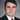# Functional vs. Object Oriented Programming

May 21, 2021

## Functional Programming Concepts

### Pure Functions

Pure functions are functions that will get the same return value given the same inputs.
for example:

``````  function square(a) {
return a ** 2
}``````

If we call `square(2)` we will always get back 4. Pure functions are easily testable because they don’t require any dependencies and we can just throw things into the input and test the output with very low overhead. The low overhead makes it really easy to test for multiple edge cases and also makes the test faster and scalable.

#### Side Effects

Side effects are things that prevent a function from returning the same output, these are “dependencies” that the function needs to know for the output.
for example:

``````  function square() {
const a = getNumberFromTheDatabase();
return a ** 2
}``````

Here we are calling square and the output is dependent on the number we get back from the database, if that number changes our output changes despite having the same input. If we wanted to test the logic in this case (squaring) we would have to stub out the function call to the database.

### Mutability

Mutability in programming is the ability to change the data.

for example:

``````  let i = 0;
i = 1;``````

The second line reassigns the variable i. Reassignment should be avoided as much as possible because it makes the code a lot harder to read. When code has a lot of reassignment its hard for the programmer to tell what a variable is at a given point.

Example of how to avoid reassignment:

Lets say you have an object of an article and you want to publish it.

``````  const article = {
text: '',
isPublished: false
}``````

You might want to use reassignment here to modify the article object, but trying to avoid reassignment how can we do this functionally?

``````  const article = {
text: '',
isPublished: false
}

function publishArticle(a) {
return {...a, isPublished: true }
}

const publishedArticle = publishArticle(article);``````

Here instead of modifying the article object we are creating a new object with isPublished set to true. Creating new data to change data is very common in functional programming. Elixir does this a lot since it is immutable.

### First Class Functions

First class functions are when functions are treated just like any other variable.

for example lets look at the new arrow syntax.

``````  const square = (a) => {
return a ** 2
}``````

The left side of this assignment looks just like any other assignment we would do for a number or an array. First class functions also means that you can pass functions as variables to other functions.

For Example:

``````  const callback = () => {
console.log('Hi');
}

const greet = (name, cb) => {
cb();
console.log(name);
}
greet('you', callback);``````

Here we are passing in the callback function as a parameter to the greet function just like any other variable.

### Lambda

A lambda in programming is an anonymous function meaning it doesn’t have a name. Usually these lambdas are passed into other functions.

To reference our previous example:

``````  const greet = (name, cb) => {
cb();
console.log(name);
}
greet('you', () => {
console.log('Hi')
});``````

The cb parameter is an anonymous function. Lambdas provide a lot of flexibilty because it allows the caller to control what its doing. For example lets look at the map function.

``````[1,2,3,4,5].map(x => x + 2);

output: [3, 4, 5, 6, 7]``````

This map function takes an anonymous function that performs operations on a given array and returns the new array.

## Object Oriented Programming Concepts

### Encapsulation

Encapsulation is the concept that objects have data and functions that modify that data. These objects are encapsulated because the outside world can’t directly modify that data without using the functions that act as an interface to the data.

### Inheritance

Inheritance allows us to extend classes creating a hierarchy. For example we might have an Animal class and a Monkey class would be an extension of that Animal class. There are common properties that animals have like height and weight, but there are also some specific properties that different monkeys have.

### Polymorphism

Polymorphism is the ability to use the same interface or often times function to represent many different types.